Plots and Results, May, 2001

On this page, you will find the results and plots of CkmFitter as of May, 2001 (the complete compendium is in our article hep-ph/0104062.). The inputs are given in this table.

 The most relevant constraints (no errors shown) in the large (rhobar,etabar) plane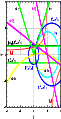eps Sketch of the unitarity triangle in the (rhobar,etabar) plane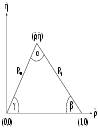eps Confidence levels for the individual constraints in the (rhobar,etabar) plane with with full errors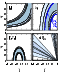eps   ps Confidence levels in the (rhobar,etabar) plane obtained from the global fit. The constraint from sin2beta is not included in the fit.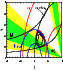eps   ps Confidence levels in the large (rhobar,etabar) plane obtained from the global fit. The constraint from sin2beta is not included in the fit.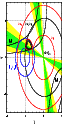eps Confidence levels in the (rhobar,etabar) plane obtained from the global fit. The constraint from sin2beta is included in the fit.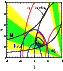eps   ps Confidence levels in the (sin2alpha,sin2beta) plane obtained from the global fit. The constraint from sin2beta is not included in the fit.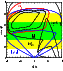eps   ps Confidence levels in the (sin2alpha,gamma) plane obtained from the global fit. The constraint from sin2beta is not included in the fit.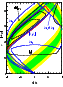eps   ps Confidence levels in the (sin2beta,gamma) plane obtained from the global fit. The constraint from sin2beta is not included in the fit.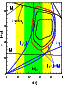eps   ps One-dimensional confidence levels for the Wolfenstein Parameters, the jarlskog parameter and the unitarity triangle angles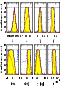eps   ps One-dimensional confidence levels for sin2beta. Shown are the results from the direct measurements and the indirect CkmFitter constraints using Rfit and Bayesian statistics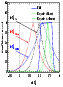eps   ps Table of all input measurements and parameters used in the global CKM fits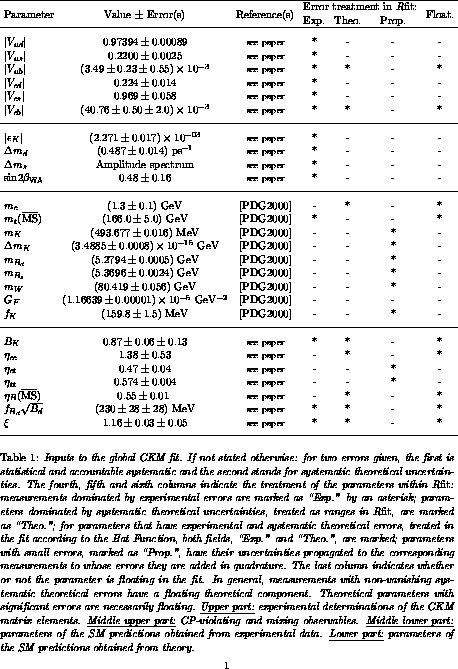ps Numerical results obtained from a global CKM fit using Rfit for the CKM parameters, the UT angles, rare B and K decays and theoretical parameters, not-including sin2beta in the fit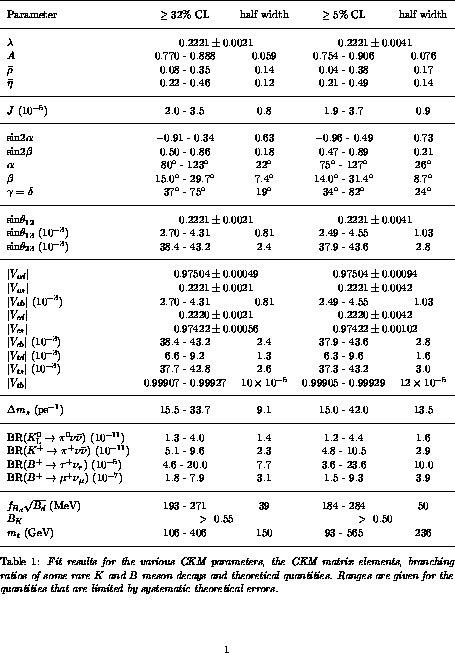ps Numerical results obtained from a global CKM fit using Rfit for the CKM parameters, the UT angles, rare B and K decays and theoretical parameters, including sin2beta in the fit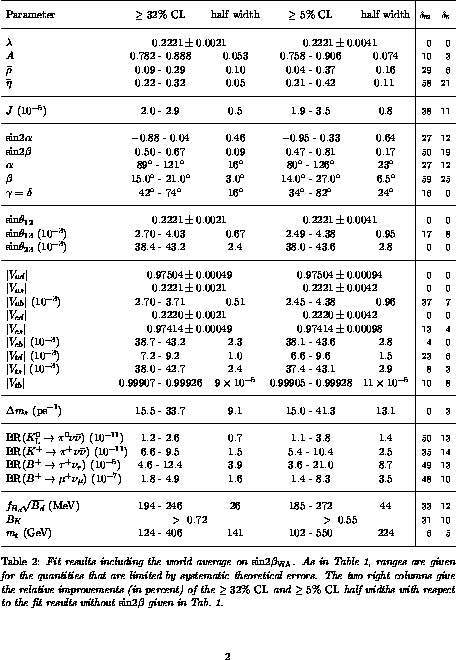ps Numerical results obtained from a global CKM fit using Bayesian statistics with Gaussian and uniform PDF's, compared to Rfit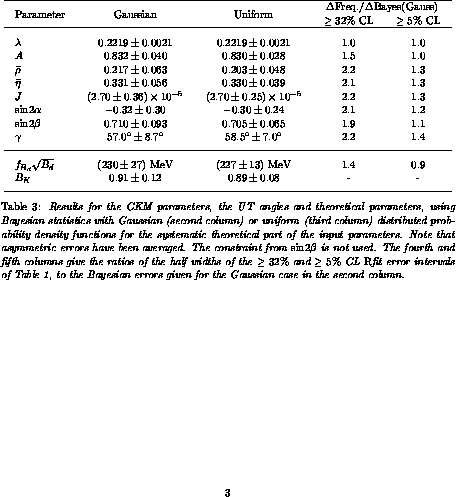ps